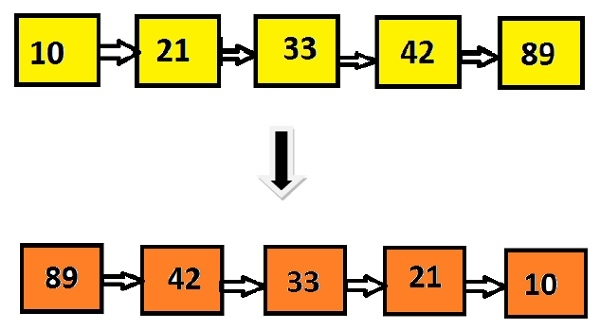# Print reverse of a Linked List without extra space and modification in C Program.

The task is to print the nodes starting from the end of the linked list without using extra space which means there shouldn’t be any extra variable instead the head pointer pointing the first node will be moved.

## Example

Input: 10 21 33 42 89
Output: 89 42 33 21 10There can be many solutions to print the linked list in reverse order, like recursive approach (use extra space), reverse the linked list(requires modification in the given Linked List), pushing the elements on a stack and then pop and display the elements one by one(requires space O(n)), but these solutions seem to be using more space than the O(1).

To achieve the result without using more than O(1) we can −

• Count the number of nodes in a linked list
• Loop from i = n to 1 and print the i-th location’s node.

## Algorithm

START
Step 1 -> create node variable of type structure
Declare int data
Declare pointer of type node using *next
Step 2 ->Declare function int get(struct node* head)
Declare variable as int count=0
Loop While newme!=NULL
Increment count by 1
Set newme = newme->next
End
Return count
Step 3 -> Declare Function void push(node** headref, char newdata)
Allocate memory using malloc
Set newnode->data = newdata
Step 4 -> Declare function int getN(struct node* head, int n)
Declare struct node* cur = head
Loop for int i=0 and i<n-1 && cur != NULL and i++
Set cur=cur->next
End
Return cur->dataStep 5 -> Declare function void reverse(node *head)
Loop For int i=n and i>=1 and i—
End
Step 6 ->In Main()
Create list using node* head = NULL
STOP

## Example

#include<stdio.h>
#include<stdlib.h>
//node structure
struct node {
int data;
struct node* next;
};
void push(struct node** headref, int newdata) {
struct node* newnode = (struct node*) malloc(sizeof(struct node));
newnode->data = newdata;
}
int count = 0;
while (newme != NULL){
count++;
newme = newme->next;
}
return count;
}
int getN(struct node* head, int n) {
for (int i=0; i<n-1 && cur != NULL; i++)
cur = cur->next;
return cur->data;
}
for (int i=n; i>=1; i--)
}
int main() {
struct node* head = NULL; //create a first node
push(&head, 89); //pushing element in the list
}
89 42 33 21 10